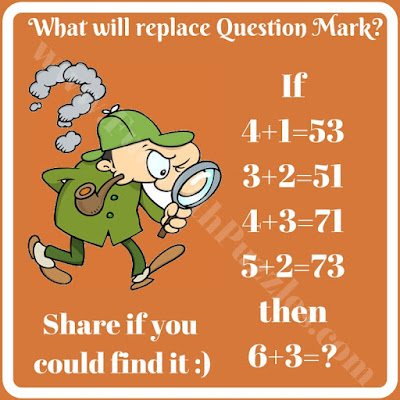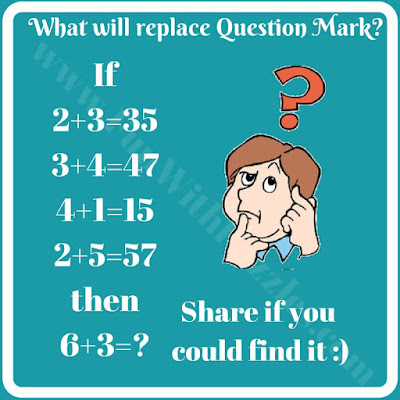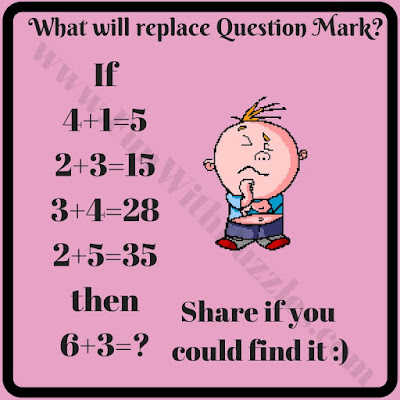Do you like to solve mathematical puzzles? There are many different logical puzzles that can be created which will require mathematical calculations to solve it. One of these puzzle types is mathematical logical equations. In these puzzles, there are some mathematical equations. These math equations are not correctly mathematical and it looks that these equations do not make any sense. However, if we study these equations logically then these equations will make full sense. So here are some brain teasers in which one has to think both logically as well as mathematically.

## How to Solve Mathematical Logical Puzzles

In these brain teasers, some Math equations are given. These Maths equations are correct logically and follow some certain pattern. One has to find the pattern followed in the given four equations and then solve the last equation correctly.
Answers to these brain teasers will be provided at the end of the post.1. Fun Math Logic Number Puzzle for Kids2. Easy Math Logic Puzzle Question for Kids3. Mathematical Thinking Puzzle Question for Teens4. Logical Thinking Brain Teaser for Teens5. Tricky Mathematical Logical Puzzle for Teens

Unknown said...

1) 39
2) 27
3) 936
4) 21